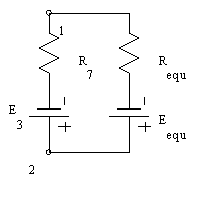Using Circuit Magic to calculate Norton Thévenin's & Norton's equivalents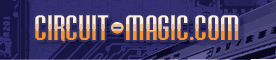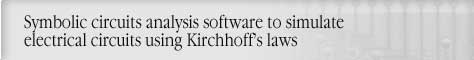Circuit analysis Tutorial
AKNM Circuit Magic- circuit analysis software

# Using Circuit Magic to calculate Norton Thévenin's & Norton's equivalents

Sample circuit shown below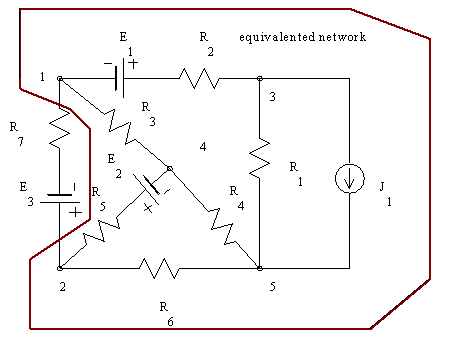Step 1. Remove branch connecting node 1 and node 2.,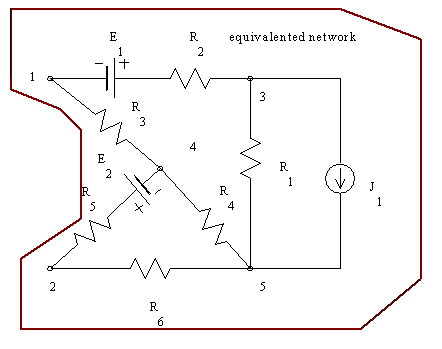Step 2 Solve circuit using Node Voltage method.

Result shown below.

V1=-10

V2=0

V3=-5

V4=-5

V5=0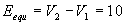V

Step 3. Resistance calculation. Remove All DC Voltage sources and branches with current sources. And connect node 1 and node 2 using the branch with current source (current =1 A). (as shown below)Step 4 Solve circuit using node voltage method.

V1=-7,2727

V2=4,5455

V3=-3,6364

V4=-0,90909

V5=0

Step 5. Calculate resistance using Ohms law.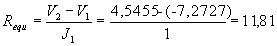ohms

Thévenin's equivalent